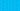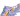PricingResources

# XIRR vs. IRR: What’s the Difference?

Written by

IRR and XIRR are Excel functions that you can use to determine the rate of return of any particular investment or project. Both functions use the same cash flow, but in many cases, they’ll produce different results. Why is this? In our guide to the difference between IRR and XIRR, we’ll take you through the ins and outs of the IRR and XIRR formulas, providing you with a comprehensive overview of XIRR vs. IRR for business accounting.

## What is the IRR formula?

IRR stands for Internal Rate of Return. Essentially, the IRR formula enables you to calculate the rate of return on an investment or project, while excluding external factors. The IRR formula is as follows:

This may be a little more complex than the accounting formulas you’ve used before, but once you know what’s going on, it’s relatively simple to navigate. Basically:

• Ct = Net Cash Inflow During Period t

• C0 = Initial Investment Cost

• IRR = Internal Rate of Return

• t = Number of Time Periods

Essentially, the IRR formula equates the net present value (NPV) of future cash flows to zero (0). This means that if you calculate the project/investment’s NPV – using IRR as the discount rate – and subtract the value of the original investment, the NPV will be equal to zero. Confused? Don’t worry too much! While you can work out the IRR of a project manually, if you so choose, most people will simply use Excel, as it’s far more efficient.

## What is the XIRR formula?

XIRR stands for Extended Internal Rate of Return. In contrast to IRR, the XIRR formula provides you with an extended rate of return that takes into account cash flows and discount rates, as well as the corresponding dates, providing you with a more accurate ROI percentage. In practical terms, if you’re working in Excel, you’ll simply need to enter two series in the XIRR formula – the series of cash flows and the corresponding dates of these cash flows.

## XIRR vs. IRR: example

Let’s look at a quick example of the IRR formula and the XIRR formula in action to understand the differences between the two Excel functions.

Imagine that Company A has made an investment in a project:

 A B C 1 Particulars Date Cash flow 2 Initial Investment 10-April-2020 -250,000 3 Return – 1st Installment 10-April-2021 100,000 4 Return – 2nd Installment 5-November-2021 150,000 5 Return – 3rd Installment 2-April-2022 125,000 6 Return – 4th Installment 17-December-2022 210,000

Using this data, we can calculate the ROI percentage in Excel.

• For the IRR formula, simply type “=IRR(C2:C6)”.

• For the XIRR formula, simply type “=XIRR(C2:C6, B2:B6)”.

This produces the following values:

• IRR = 40%

• XIRR = 58%

As you can see, despite the two formulas using the same cash flows, they have produced different results.

## What’s the difference between IRR and XIRR?

As we’ve explained, the key difference between IRR and XIRR is the way each formula handles cash flows. IRR doesn’t take into account when the actual cash flow takes place, so it rolls them up into annual periods. By contrast, the XIRR formula considers the dates when the cash flow actually happens. Because of this, XIRR is a more accurate way to evaluate an investment. So, when it comes to XIRR vs. IRR, you should always use XIRR unless you explicitly want to calculate the IRR over a yearly period.

## We can help

GoCardless helps you automate payment collection, cutting down on the amount of admin your team needs to deal with when chasing invoices. Find out how GoCardless can help you with ad hoc payments or recurring payments.## Interested in automating the way you get paid? GoCardless can help

Contact sales

Sales

Contact Sales

+1(415) 523-2279

Support

help@gocardless.com

+1 (628) 241-0044